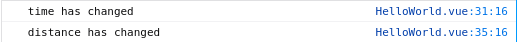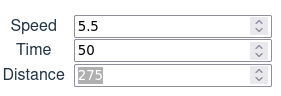# Binding multiple values together in Vue.js

Thanks to Vue.js reactivity, updating a value when another value has changed is really simple.

Imagine you have a form in which you prompt for speed and time, it's super easy to have the distance update automatically:

``````import { computed, defineComponent, ref } from 'vue';

export default defineComponent({
setup() {
const speed = ref(0);
const time = ref(0);

const distance = computed(() => speed.value * time.value);

return { speed, time, distance };
},
});``````

In this code block, the `distance` value is automagically computed from `speed` and `time` values.

Even though it's not the recommended way, we could achieve the same by using a watcher:

``````import { defineComponent, ref, watch } from 'vue';

export default defineComponent({
setup() {
const speed = ref(0);
const time = ref(0);
const distance = ref(0);

watch([speed, time], ([new_speed, new_time]) => {
distance.value = new_speed * new_time;
});

return { speed, time, distance };
},
});``````

This works great but it's a one-way flow.

How could we update this code to have any value update trigger the two other values updates?

## The problematic solution #

First of all, let's also watch for `distance` value changes:

``````watch(
[speed, time, distance],
(
[new_speed, new_time, new_distance],
[old_speed, old_time, old_distance],
) => {
// TODO
},
);``````

In this update we're:

• watching the 3 values
• getting the current state (`new_*` variables)
• getting the previous state (`old_*` variables)

The current & previous states will help us identify what has changed:

``````watch(
[speed, time, distance],
(
[new_speed, new_time, new_distance],
[old_speed, old_time, old_distance],
) => {
if (new_speed !== old_speed) {
console.log('speed has changed');
} else if (new_time !== old_time) {
console.log('time has changed');
} else {
console.log('distance has changed');
}
},
);``````

We could then update the third value from the two others:

``````watch(
[speed, time, distance],
(
[new_speed, new_time, new_distance],
[old_speed, old_time, old_distance],
) => {
if (new_speed !== old_speed) {
console.log('speed has changed');
distance.value = new_speed * time.value;
} else if (new_time !== old_time) {
console.log('time has changed');
distance.value = speed.value * new_time;
} else {
console.log('distance has changed');
time.value = new_distance / speed.value;
}
},
);``````

This code will give you the expected results but… If you look at the console, you'll see that the watcher triggers itsef in a loop:

With a single change, you get two runs of the watcher:It's getting even worse if you decide, for example, that you'll round the result of the calculations:

``````watch(
[speed, time, distance],
(
[new_speed, new_time, new_distance],
[old_speed, old_time, old_distance],
) => {
if (new_speed !== old_speed) {
console.log('speed has changed');
distance.value = Math.round(new_speed * time.value);
} else if (new_time !== old_time) {
console.log('time has changed');
distance.value = Math.round(speed.value * new_time);
} else {
console.log('distance has changed');
time.value = Math.round(new_distance / speed.value);
}
},
);``````

If you increase the distance when speed is not a round value, then you'll get weird results:Increasing the distance one by one will give you the following sequence: 275, 276, 277, 281. There are values you can't reach!

That's because the distance value gets re-computed when the time value gets computed itself.

## A better solution #

How could we get these three values updated without triggering loops?

The answer is that we'll need to stop the watcher from watching while we update one of the values.

First, let's extract the update logic into a function:

``````function update_values(
[new_speed, new_time, new_distance]: number[],
[old_speed, old_time, old_distance]: number[],
) {
// The function contains what was in the `watch(..., (...) => {...})` statement before
}
watch([speed, time, distance], update_values);``````

Then get the watcher handle into a variable:

``````let unwatch: ReturnType<typeof watch>;
function update_values(
[new_speed, new_time, new_distance]: number[],
[old_speed, old_time, old_distance]: number[],
) {
// The original contents of the function has not changed here
}
unwatch = watch([speed, time, distance], update_values);``````

Finally stop the watcher when entering the `update_values` function and recreate it before leaving:

``````let unwatch: ReturnType<typeof watch>;
function update_values(
[new_speed, new_time, new_distance]: number[],
[old_speed, old_time, old_distance]: number[],
) {
// Stop the watcher
unwatch();

// The original contents of the function has not changed here

// Recreate the watcher
unwatch = watch([speed, time, distance], update_values);
}
unwatch = watch([speed, time, distance], update_values);``````

## Conclusion #

With this implementation, the watcher gets triggered only once, whatever the value that is changed and whatever the feedback that the update may generate on the value that originally changed.

It makes the code more efficient and more robust. You'd probably spend hours or days trying to find out where the bug is once in production.

Finally this pattern prevents resource wasting on the user computer and your application will appear to be more fluent, making it a better experience.